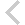FICO Xpress Optimization Examples Repository
 FICO Optimization Community FICO Xpress Optimization HomeIntroductory examples

Description
approx burglar chess Problem name and type, features Difficulty Approximation: Piecewise linear approximation ** SOS-2, Special Ordered Sets, piecewise linear approximation of a nonlinear function MIP modeling: Knapsack problem: 'Burglar' * simple MIP model with binary variables, data input from text data file, array initialization, numerical indices, string indices, record data structure LP modeling: Production planning: 'Chess' problem * simple LP model, solution output, primal solution values, slack values, activity values, dual solution values All item discount pricing: Piecewise linear function *** SOS-1, Special Ordered Sets, piecewise linear function, approximation of non-continuous function, step function Incremental pricebreaks: Piecewise linear function *** SOS-2, Special Ordered Sets, piecewise linear function, step function

Further explanation of this example: 'Applications of optimization with Xpress-MP', Introductory examples (Chapters 1 to 5) of the book 'Applications of optimization with Xpress-MP'

Source Files

Data Files

pricebrinc.mos

```(!******************************************************
Mosel Example Problems
======================

file pricebrinc.mos
```````````````````
Incremental pricebreaks formulated with SOS2

(c) 2008 Fair Isaac Corporation
author: S. Heipcke, Sep. 2006
*******************************************************!)

model "Incremental pricebreaks (SOS2)"
uses "mmxprs"

declarations
NB = 3                                ! Number of price bands
BREAKS = 0..NB
COST: array(1..NB) of real            ! Cost per unit within price bands
w: array(BREAKS) of mpvar             ! Weight variables
x: mpvar                              ! Total quantity bought
B,CBP: array(BREAKS) of real          ! Break points, cost break points
end-declarations

DEM:= 150                              ! Demand
B::  [0, 50, 120, 200]
COST:: [0.8, 0.5, 0.3]

CBP(0):= 0
forall(i in 1..NB) CBP(i):= CBP(i-1) + COST(i) * (B(i)-B(i-1))

! Objective: total price
TotalCost:= sum(i in BREAKS) CBP(i)*w(i)

! Meet the demand
x = DEM

! Definition of x
Defx:= x = sum(i in BREAKS) B(i)*w(i)

! Weights sum up to 1
sum(i in BREAKS) w(i) = 1

! Definition of SOS2
! (we cannot use 'is_sos2' since there is a 0-valued coefficient)
makesos2(union(i in BREAKS) {w(i)}, Defx)

! Solve the problem
minimize(TotalCost)

! Solution printing
writeln("Objective: ", getobjval,
" (price per unit: ", getobjval/DEM, ")")
forall(i in BREAKS)
writeln("w(", i, "): ", getsol(w(i)), " (price per unit: ",
if(i>0, CBP(i)/B(i), CBP(i)), ")")

end-model

```